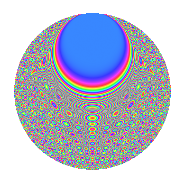# Properties

 Label 1350.2.bdLevel 1350 Weight 2 Character orbit bd Rep. character $$\chi_{1350}(17,\cdot)$$ Character field $$\Q(\zeta_{60})$$ Dimension 480 Sturm bound 540

# Related objects

## Defining parameters

 Level: $$N$$ = $$1350 = 2 \cdot 3^{3} \cdot 5^{2}$$ Weight: $$k$$ = $$2$$ Character orbit: $$[\chi]$$ = 1350.bd (of order $$60$$ and degree $$16$$) Character conductor: $$\operatorname{cond}(\chi)$$ = $$225$$ Character field: $$\Q(\zeta_{60})$$ Sturm bound: $$540$$

## Dimensions

The following table gives the dimensions of various subspaces of $$M_{2}(1350, [\chi])$$.

Total New Old
Modular forms 4512 480 4032
Cusp forms 4128 480 3648
Eisenstein series 384 0 384

## Trace form

 $$480q + O(q^{10})$$ $$480q - 60q^{16} - 12q^{20} - 24q^{23} - 48q^{25} + 24q^{37} + 36q^{38} + 48q^{47} + 48q^{50} + 24q^{55} - 12q^{58} + 60q^{59} - 24q^{65} + 12q^{67} + 144q^{68} + 432q^{77} + 48q^{82} + 60q^{83} + 24q^{85} - 24q^{92} + 60q^{95} + 36q^{97} + O(q^{100})$$

## Decomposition of $$S_{2}^{\mathrm{new}}(1350, [\chi])$$ into newform subspaces

The newforms in this space have not yet been added to the LMFDB.

## Decomposition of $$S_{2}^{\mathrm{old}}(1350, [\chi])$$ into lower level spaces

$$S_{2}^{\mathrm{old}}(1350, [\chi]) \cong$$ $$S_{2}^{\mathrm{new}}(225, [\chi])$$$$^{\oplus 4}$$$$\oplus$$$$S_{2}^{\mathrm{new}}(450, [\chi])$$$$^{\oplus 2}$$$$\oplus$$$$S_{2}^{\mathrm{new}}(675, [\chi])$$$$^{\oplus 2}$$

## Hecke Characteristic Polynomials

There are no characteristic polynomials of Hecke operators in the database